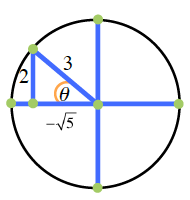### Home > PC > Chapter 4 > Lesson 4.3.1 > Problem4-123

4-123.

Given sin θ =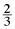and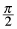< θ <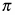. Find the values of the 5 other trigonometric functions. Homework Help ✎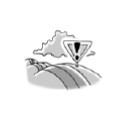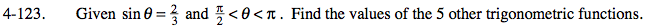1. Draw a non-unit circle placing in the triangle in the appropriate quadrant.
2. Label/find the sides of the triangle. Don't forget the check the sign.
3. Now the other 5 trig functions can be easily found from these sides.

x2 + 22 = 32
x2 = 5

$x=\pm\sqrt{5}$

In this case we are in the 2nd
quadrant so it must be negative.